•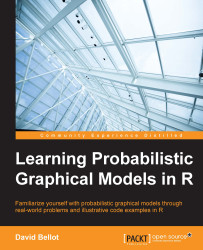#### Learning Probabilistic Graphical Models in R#### Overview of this book

Probabilistic graphical models (PGM, also known as graphical models) are a marriage between probability theory and graph theory. Generally, PGMs use a graph-based representation. Two branches of graphical representations of distributions are commonly used, namely Bayesian networks and Markov networks. R has many packages to implement graphical models. We’ll start by showing you how to transform a classical statistical model into a modern PGM and then look at how to do exact inference in graphical models. Proceeding, we’ll introduce you to many modern R packages that will help you to perform inference on the models. We will then run a Bayesian linear regression and you’ll see the advantage of going probabilistic when you want to do prediction. Next, you’ll master using R packages and implementing its techniques. Finally, you’ll be presented with machine learning applications that have a direct impact in many fields. Here, we’ll cover clustering and the discovery of hidden information in big data, as well as two important methods, PCA and ICA, to reduce the size of big problems.
Learning Probabilistic Graphical Models in RCreditswww.PacktPub.comPrefaceFree Chapter
Probabilistic ReasoningExact InferenceLearning ParametersBayesian Modeling – Basic ModelsApproximate InferenceBayesian Modeling – Linear ModelsProbabilistic Mixture ModelsAppendixIndexWhen computing the distribution of one variable (or a subset of variables), the main operation is the marginalization, which consists of summing over a variable (or a subset of variables) to eliminate it from the main expression. If we call ϕ a factor in the factorization of the joint distribution, we can use the following properties to generalize and improve the variable elimination algorithm we saw in the previous section:

• Commutativity: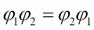• Associativity: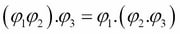• And if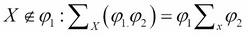If again we apply it to the joint distribution P(ABCD) from the previous section, we again obtain: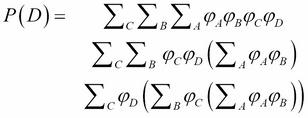In the end, the main expression that comes again and again is the sum-product over a factor, which can be written as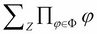.

So in general, if we can find a good ordering of the factors or the variables in the case of a directed graphical model as we saw until now, we can, by applying the previous sum-product formula, eliminate step by step each variable until we obtain the desired subset.

The ordering must marginalize...